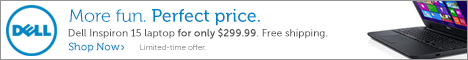## Pages## Monday, September 18, 2006

### How to Calculate APY

Have you ever wondered how banks calculate the annual percentage yield (APY) of a bank account? Suppose that an account pays 5.84% (nominal rate) compounded daily and yields 6.01% APY. The APY is the annual percentage yield, and is the best number to use when comparing rates from different banks. To calculate the APY from the nominal rate, you will need a scientific or financial calculator. A computer spreadsheet could be used instead of a calculator.

Warning: math is involved in the next section. In this example,

1) Enter the interest rate in decimal form: 0.0584
2) Divide the rate by 365 (number of days in a year)
3) Add 1 to the result
4) Then use the y^x key, and type 365 for the number of days.

You should end up with something that says 1.060134.... The digits after the decimal point represent the APY. In this case, it is 6.01% APY.

Shortcut: In most cases, you can take the nominal interest rate: 0.0584, and hit the e^x key on your calculator to get 1.060138.... This quickly approximates the APY, assuming that interest is compounded daily.

If you have an account that is compounded monthly, then replace the 365's above with 12 (number of months in a year). In this case, if interest were compounded monthly, then the APY would round off to 6.00% APY.

pfstock